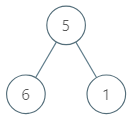1120. Maximum Average Subtree

Given the `root` of a binary tree, find the maximum average value of any subtree of that tree.

(A subtree of a tree is any node of that tree plus all its descendants. The average value of a tree is the sum of its values, divided by the number of nodes.)

Example 1:```Input: [5,6,1]
Output: 6.00000
Explanation:
For the node with value = 5 we have an average of (5 + 6 + 1) / 3 = 4.
For the node with value = 6 we have an average of 6 / 1 = 6.
For the node with value = 1 we have an average of 1 / 1 = 1.
So the answer is 6 which is the maximum.
```

Note:

1. The number of nodes in the tree is between `1` and `5000`.
2. Each node will have a value between `0` and `100000`.
3. Answers will be accepted as correct if they are within `10^-5` of the correct answer.

1120. Maximum Average Subtree
``````struct Solution;
use rustgym_util::*;

trait Postorder {
fn postorder(&self, max: &mut f64) -> (i32, i32);
}

fn postorder(&self, max: &mut f64) -> (i32, i32) {
let res = if let Some(node) = self {
let val = node.borrow().val;
let (sum_l, size_l) = node.borrow().left.postorder(max);
let (sum_r, size_r) = node.borrow().right.postorder(max);
(sum_l + sum_r + val, size_l + size_r + 1)
} else {
(0, 0)
};
*max = (*max).max(res.0 as f64 / res.1 as f64);
res
}
}

impl Solution {
fn maximum_average_subtree(root: TreeLink) -> f64 {
let mut res = std::f64::MIN;
root.postorder(&mut res);
res
}
}

#[test]
fn test() {
use assert_approx_eq::assert_approx_eq;
let root = tree!(5, tree!(6), tree!(1));
let res = 6.0;
assert_approx_eq!(Solution::maximum_average_subtree(root), res);
}
``````